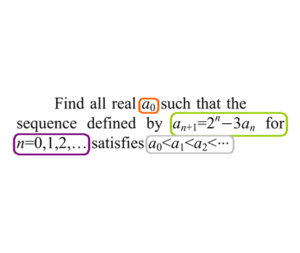Home -> Solved problems -> Challenging problem

## Challenging problem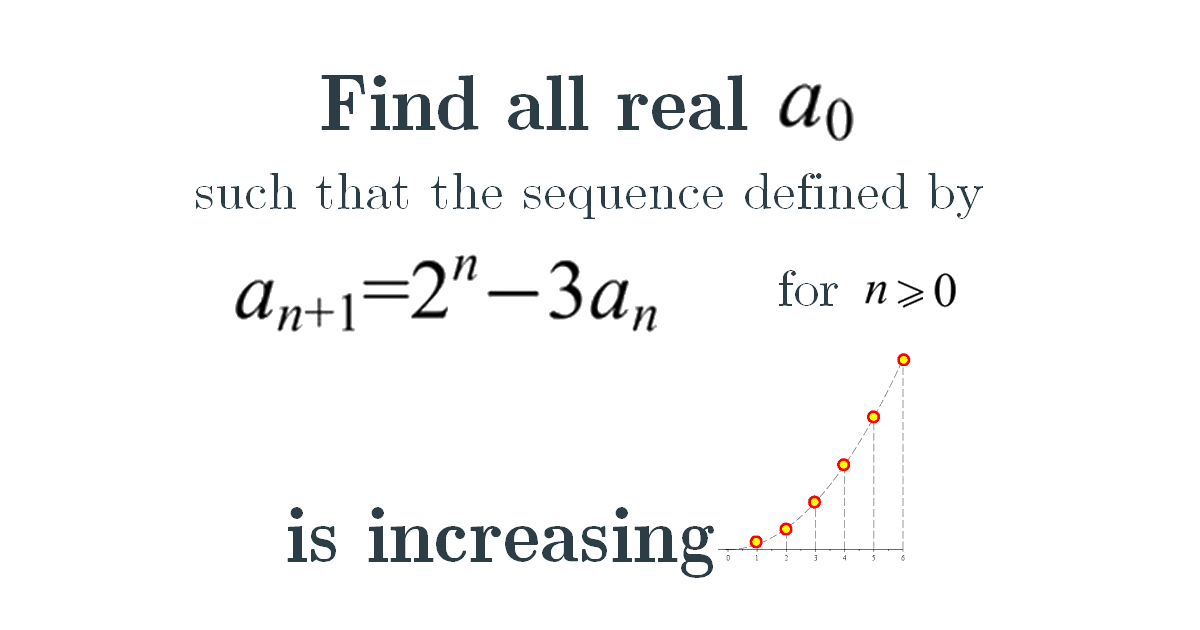### Solution

Let’s start, we have
\begin{aligned} a_{n+1} &=2^{n}-3 a_{n}=2^{n}-3 \times 2^{n-1}+3^{2} a_{n-1} \\ &=\cdots=2^{n}+\sum_{j=1}^{n}(-3)^{j} 2^{n-j}+(-3)^{n+1} a_{0} \\ &=\frac{2^{n+1}}{5}+\left(a_{0}-\frac{1}{5}\right)(-3)^{n+1} \end{aligned}

If $$a_{0}=\frac{1}{5}$$, then we have no problem. If $$a_{0}\neq\frac{1}{5}$$, $$\left(\frac{2}{3}\right)^{n}$$ goes to 0 when $$n\rightarrow\infty$$, so $$\frac{a_{n}}{3^{n}}$$ will have the same sign as $$\left(a_{0}-\frac{1}{5}\right)\left(-1\right)^{n}$$ when $$n$$ is large. Hence, $$a_{n}<a_{n+1}$$ will not hold then contradiction
Home -> Solved problems -> Challenging problem

### Related Topics

Find the volume of the square pyramid as a function of $$a$$ and $$H$$ by slicing method.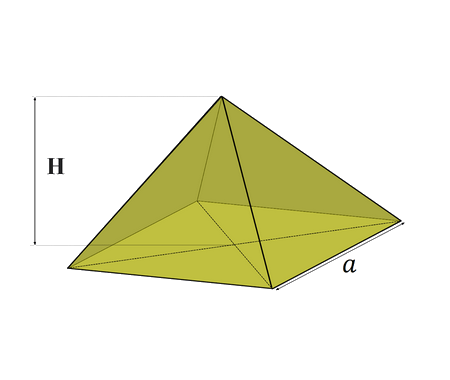Prove that $\lim_{x \rightarrow 0}\frac{\sin x}{x}=1$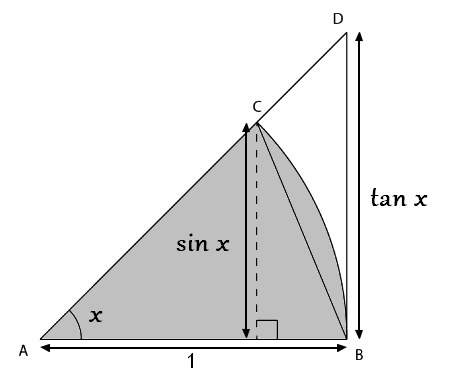Prove that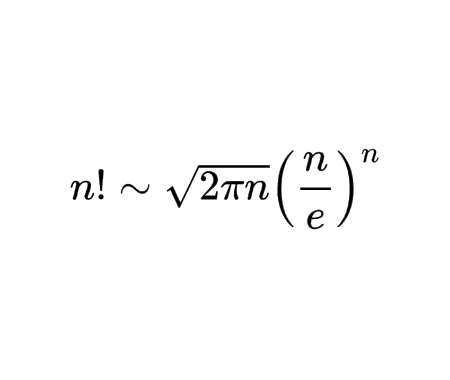Calculate the half derivative of $$x$$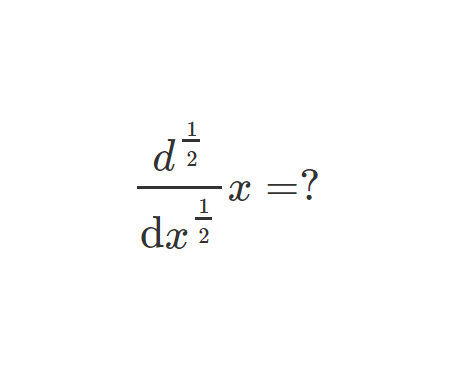Prove Wallis Product Using Integration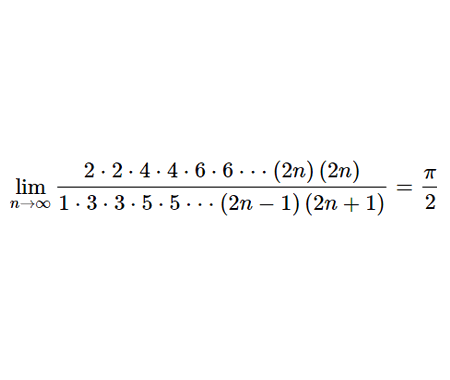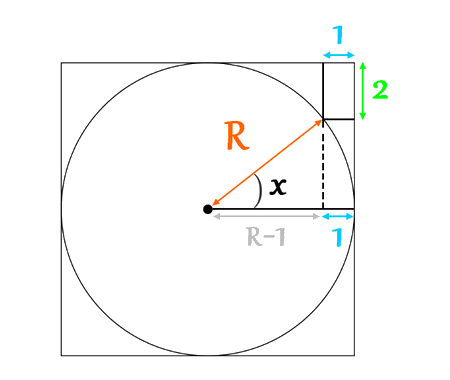Calculate the volume of Torus using cylindrical shells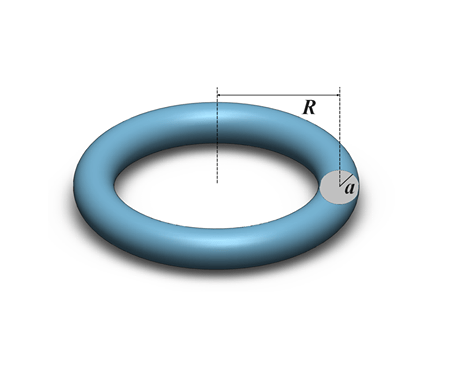Find the derivative of exponential $$x$$ from first principles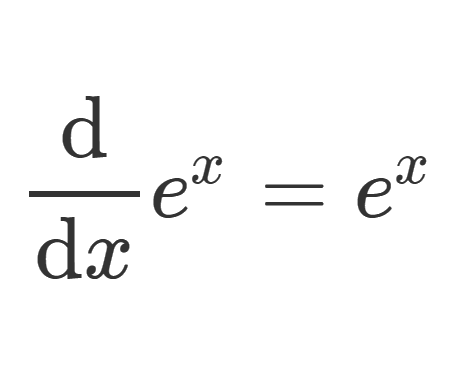Calculate the sum of areas of the three squares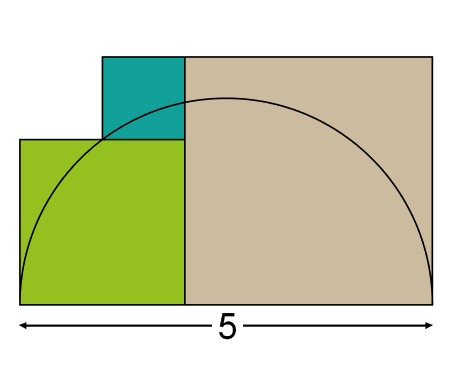Find the equation of the curve formed by a cable suspended between two points at the same height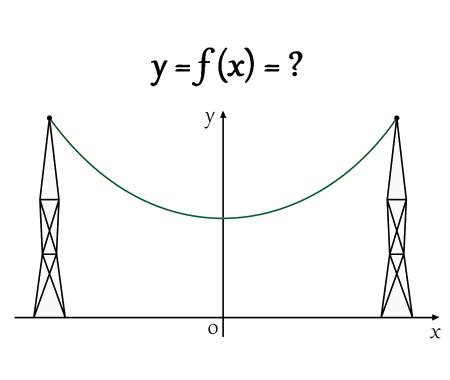Solve the equation for real values of $$x$$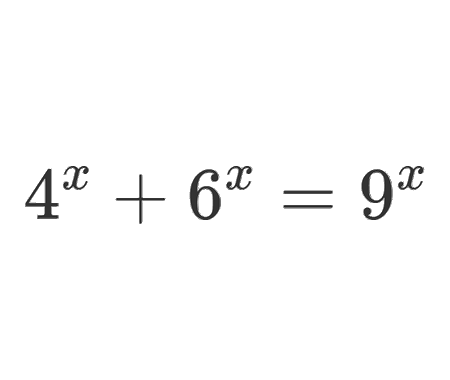Solve the equation for $$x\epsilon\mathbb{R}$$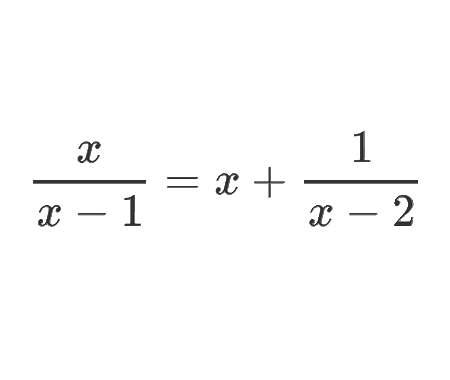Determine the angle $$x$$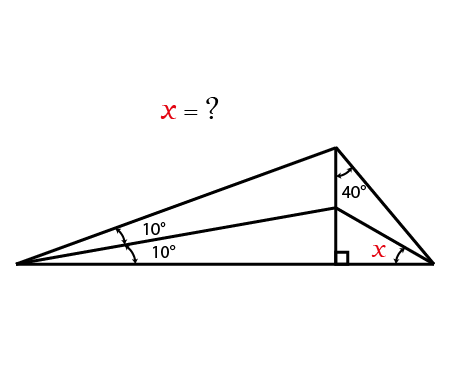Calculate the following limit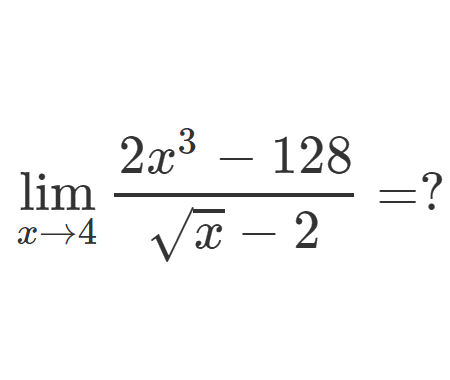Calculate the following limit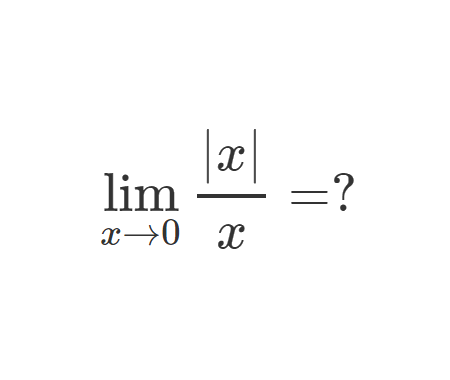Calculate the integral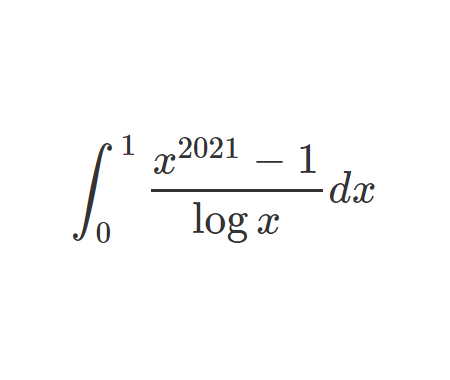Challenging problem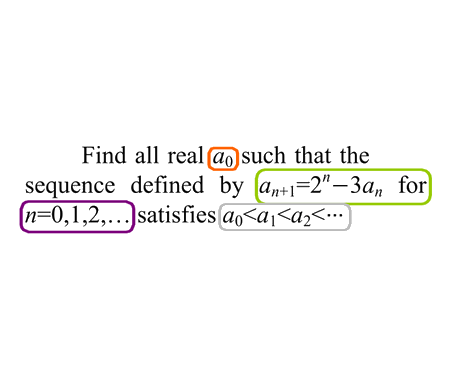Home -> Solved problems -> Challenging problem

#### Share the solution: Challenging problem